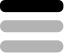OperatingSystems / Operating System Management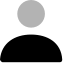Luca king 7 studios.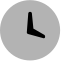4 months ago

## Maths calculator.

#### Teaser

Perform complex math calculations with ease using [BEST_PROMPT]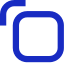#### Prompt Hint

- Complex numbers - Matrices and determinants - Calculus (differentiation and integration) - Graphing and plotting equations - Statistical analysis - Probability distributions - Financial functions (e.g. compound interest)#### Prompt

Learn more about the latest prompt: Maths calculator. Get the details such as Perform complex math calculations with ease using [BEST_PROMPT]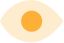1.3k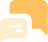2.2kTry this prompt on ChatGPT